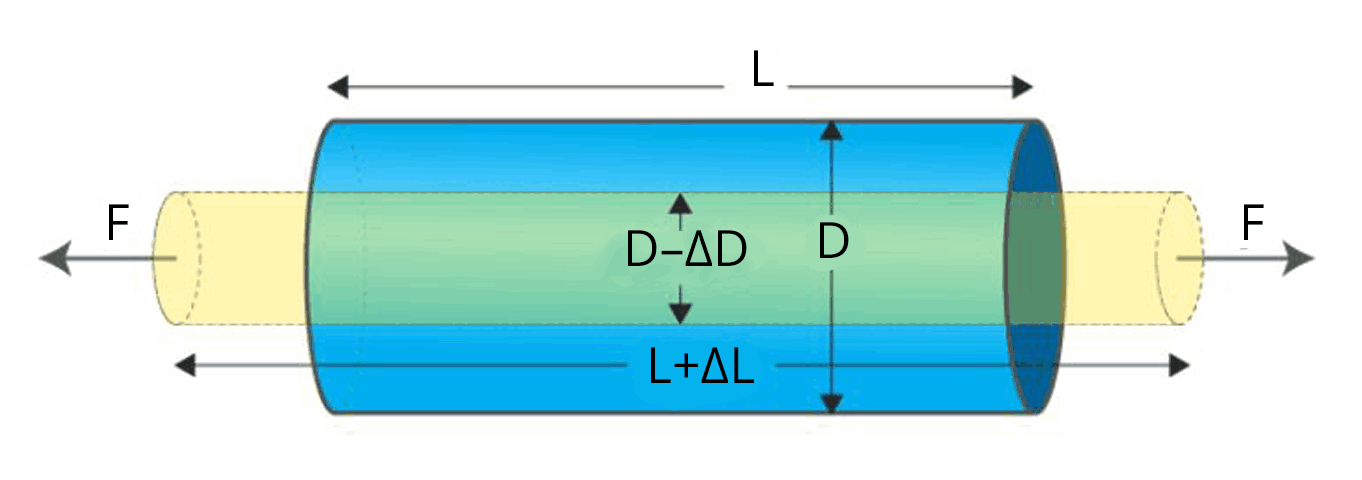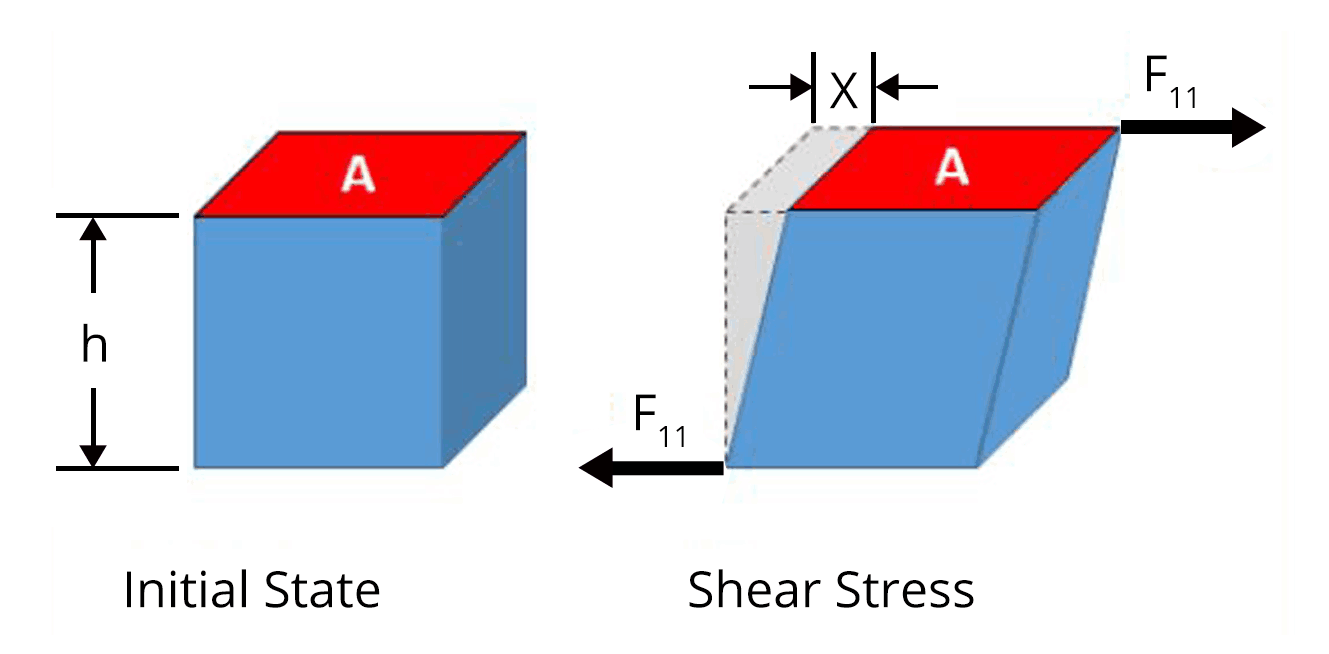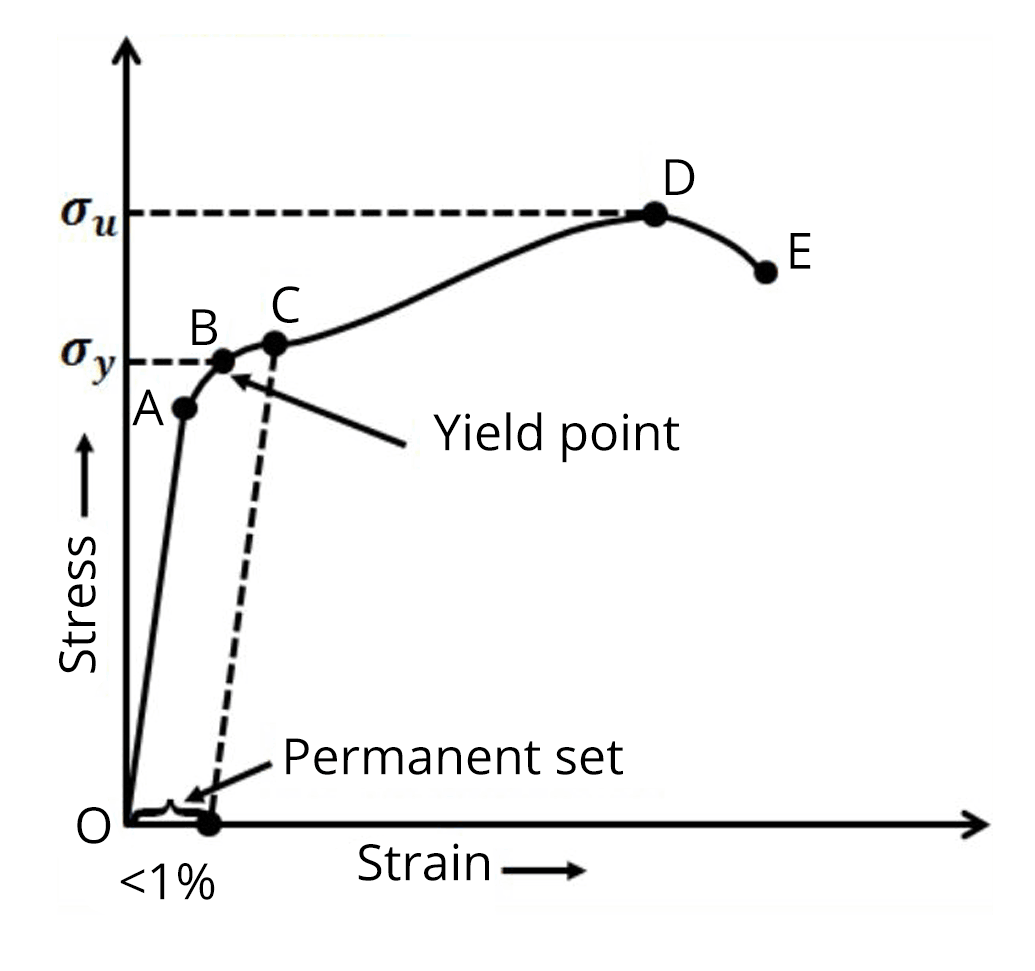# NEET Important Chapter- Mechanical Properties of Solids

Get plus subscription and access unlimited live and recorded courses## Mechanical Properties of Solids for NEET

The chapter Mechanical properties of solids is one of the easiest chapters in physics as far as the NEET exam is concerned. As the name of the chapter implies, we deal with the properties of solids and their behaviour under applied stress and some concept-oriented problems from this chapter.

In the chapter Mechanical properties of solids, first, we will learn about different types of stress like longitudinal stress and  bulk stress along with different types of strain. The stress-strain curve of a wire under longitudinal stress is studied in detail. Hooke's law and types of modulus of elasticity are very important in this chapter. Problems related to young’s modulus, bulk modulus and rigidity modulus are discussed in this chapter.

Let us see the important concepts, mechanical properties of solids formulas in this chapter Mechanical properties of solids needed for the  NEET exam with solved mechanical properties of solids numerical examples.

### Mechanical Properties of Solids- Important Topics

• Elastic behaviour of solids

• Longitudinal stress and Longitudinal strain

• Volume stress and volume strain

• Volume stress and volume strain

• Shear or tangential stress or shearing strain

• Young’s modulus

• Bulk modulus

• Modulus of rigidity

• Work done in stretching a strain

### Important Concepts of Mechanical Properties of Solids

 Name of the Concept Key Points of the Concept Elastic behaviour of solids When a deforming force is applied to a body, it changes its shape or size.Elasticity is the ability to regain its original shape and size even after deforming force is removed.When deforming force is applied, atoms are displaced from their equilibrium position and return back to the initial position due to interatomic forces after deforming force is removed.The restoring force acting per unit area of cross-section of deformed force is called stress having unit N/m2.The strain is the ratio of change in dimension to the original dimension when the deforming force is applied.$\text{stress}=\dfrac{\text{Force}}{\text{Area}}$Plasticity is the ability of a body to undergo permanent deformation even after deforming force is removed. Longitudinal stress and Longitudinal strain When a deforming force is applied parallel to the length of a rod, its length increases and the stress developed is called longitudinal stress or tensile stress.It occurs in solids only.Longitudinal strain is the ratio of change in length to the original length due to longitudinal stress.$\text{Longitudinal strain}=\dfrac{\text{change in length}}{\text{original length}}$$\text{Longitudinal strain}=\dfrac{\Delta l}{l} Volume stress and volume strain Due to volume stress or bulk stress, the volume and density of the body but the shape remains the same. (Image will be uploaded soon)The deforming force applied is normal to the surface at all points.Bulk stress occurs in solids, liquids and gases.Volume strain is the ratio of change in volume to the original volume given by the formula,\text{Volume strain}=\dfrac{\text{change in volume}}{\text{original volume}}$$\text{Longitudinal strain}=\dfrac{\Delta V}{V}$ Shear or tangential stress or shearing strain Tangential stress or shear stress is developed when the deforming force is applied tangentially to the surface.Due to shearing stress, there is the relative displacement between successive layersShearing strain is defined as the angle in radians through which the vertical surface gets turned due to tangential force and its formula is given by,$\text{Shearing strain}=\dfrac{\text{x}}{h}$ Strain-strain curve Stress-strain shows the behaviour of the wire with an increase in load.In the region from O to A, the curve is linear and obeys’ Hooke's law. The point A is called the proportional limit.According to Hooke's law, stress is proportional to strain within the proportional limit.The wire shows elastic behaviour up to point B and point B is called the yield point or elastic limit.The stress corresponding to the yield point is called yield strength.Beyond point B, it loses its elastic behaviour and does not regain its original length even after deforming force is removed.The stress corresponding to point  D is called ultimate strength. Young’s modulus Young’s modulus is the ratio of longitudinal stress to the longitudinal strain.$\text{Young's modulus}=\dfrac{\text{Longitudinal stress}}{\text{longitudinal strain}}$$\text{Young's modulus}=\dfrac{F/A}{\Delta l/l} Bulk modulus Bulk modulus is the ratio of normal stress to the volume strain given by the formula,\text{Bulk modulus}=\dfrac{\text{Normal stress}}{\text{Volumetric strain}}$$\text{Bulk modulus}=\dfrac{F/A}{-\Delta V/V}$$\text{Bulk modulus}=\dfrac{-PV}{\Delta V} Modulus of rigidity Within the limits of proportionality, the ratio of tangential stress to the shearing strain is called modulus of rigidity\text{Rigidity modulus}=\dfrac{\text{shearing stress}}{\text{shearing strain}}$$\eta=\dfrac{F/A}{\text{x/h}}$Work done in stretching a strain Work done in stretching a wire having young’s modulus(Y) under a force (F) is given by the formula,$W=\dfrac{1}{2}\dfrac{YA\Delta l^2}{l}$

### List of Important Formulae

 Sl. No Name of the Concept Formula 1. Elongation (Δl) of a wire by its own weight(W) $W=\dfrac{Mgl}{2AY}$ 2. Thermal stress on a rod fixed between two rigid supports. $\text{Thermal stress }=Y\alpha\Delta T$Where ⍺ is the coefficient of linear expansion and ΔT is the change in temperature 3. Force constant of the wire having a length(l) and area of cross section(A) $K=\dfrac{YA}{l}$ 4. Depression produced in the beam of bridge (Image will be uploaded soon)$\delta=\dfrac{Wl^3}{4Ybd^3}$ 5. Energy stored per unit volume of wire $U_V=\dfrac{1}{2}\times\text{stress}\times\text{strain}$$U_V=\dfrac{1}{2}\times Y\times\text{strain}^2$ 6. Compressibility(K) $K=\dfrac{\Delta V}{PV}$ 7. Poisson;s ratio $\sigma=\dfrac{-\Delta r/r}{-\Delta l/l}$

### Solved Examples

1. A long elastic spring is stretched by 2 cm and its potential energy is U. If the spring is stretched by 10 cm, then the  P.E will be,

a. U

b. 98U/5

c. 3U

d. 25U

Ans:

Given, Potential energy of spring stretched by 2 cm = U

$U=\dfrac{1}{2}K(2~cm)^2$....(1)

The potential energy of the spring stretched by 10 cm can be calculated using the formula given by,

$U'=\dfrac{1}{2}K(10~cm)^2$....(2)

Divide equation (2) by equation (1) to calculate the potential energy of the spring stretched by 10 cm

$\dfrac{U'}{U}=\dfrac{\dfrac{1}{2}K(10~cm)^2}{\dfrac{1}{2}K(2~cm)^2}$

$U'=25U$

Therefore, the correct answer is option d).

Key point: The potential energy of the spring depends on the spring constant and the elongation.

1. A cube of aluminium of 0.1 m is subjected to a shearing force of 100 N? The top face of the cube is displaced through 0.02 cm with respect to the bottom face. The shearing strain will be

Ans: Given,

The height of the cube, h = 0.1 m = 10 cm

The displacement of the top face due to the tangential force of 100 N, x = 0.02 cmThe shearing strain can be calculated using the formula,

$\text{Shearing strain}=\dfrac{\text{x}}{h}$

$\text{Shearing strain}=\dfrac{\text{0.02 cm}}{10 \text{ cm}}$

$\text{Shearing strain}=0.002$

Key point:  Shearing strain is equal to the ratio of displacement of the top face to the  height of the cube.

### Previous Year Questions from NEET paper

1. When a block of mass M is suspended by a long wire of length L, the length of the wire becomes (L+l). The elastic potential energy stored in the extended  wire is (NEET 2019)

a. Mgl

b. 2Mgl

c. Mgl/2

d. Mgl/4

Ans:

The force acting on the wire is related to the extension of the wire

$F=Kl$

$K=\dfrac{F}{l}$

$K=\dfrac{Mg}{l}$....(1)

The energy stored in the wire is given by,

$U=\dfrac{1}{2}Kl^2$....(2)

Put equation (1) in equation (2)

$U=\dfrac{1}{2}\dfrac{Mg}{l}l^2$

$U=\dfrac{1}{2}Mgl$

Therefore, the correct answer is option C.

Key point:  When a wire is suspended by a mass, then weight acting on the mass acts as the deforming force.

1. The bulk modulus of a spherical object is ‘B’. If it is subjected to uniform pressure ‘p’, the fractional decrease in radius will be  (NEET 2017)

a. B/3p

b. 3p/B

c. p/3B

d. p/B

Ans:

The fraction volume of a spherical object having radius r is given by,

$V=\dfrac{4\pi r^3}{3}$

$\dfrac{\Delta V}{V}=3\dfrac{\Delta r}{r}$...(1)

The formula to calculate the bulk modulus is given by,

$B=\dfrac{p}{\Delta V/V}$

${\Delta V/V}=\dfrac{p}{B}$...(2)

Put equation(1) in equation (2) to calculate the fractional decrease in radius

${3\dfrac{\Delta r}{r}}=\dfrac{p}{B}$

${\dfrac{\Delta r}{r}}=\dfrac{p}{3B}$

Key point:  Fractional decrease in radius can be obtained from the formula for the volume of the sphere.

### Practice Questions

1. If the volume of the wire remains constant when subjected to tensile stress, then the value of Poisson's ratio of the material of the wire is,

(Ans: 0.5 )

2. What increase in pressure is required to decrease the volume of 200 litres of water by 0.004 per cent? Given bulk modulus of water is 2100 MPa

(Ans: 84 KPa)

### Conclusion

In this article, we discussed important topics, and important formulas  in the mechanical properties of solids chapter from the NEET point of view. Students must make sure that they do not miss any of the above important topics to obtain a good score on the NEET  exam.

See More## NEET Important Dates

View All Dates
NEET 2022 exam date and revised schedule have been announced by the NTA. NEET 2022 will now be conducted on 17-July-2022, and the exam registration closes on 20-May-2022. You can check the complete schedule on our site. Furthermore, you can check NEET 2022 dates for application, admit card, exam, answer key, result, counselling, etc along with other relevant information.
See More
View All Dates## NEET Information

Application Form
Eligibility Criteria
Reservation Policy
NTA has announced the NEET 2022 application form release date on the official website https://neet.nta.nic.in/. NEET 2022 Application Form is available on the official website for online registration. Besides NEET 2022 application form release date, learn about the application process, steps to fill the form, how to submit, exam date sheet etc online. Check our website for more details.## NEET 2022 Study Material

View NEET Syllabus in Detail
View NEET Syllabus in Detail## NEET 2022 Study Material

View all study material for NEET
All
Physics
Chemistry
Biology
See All## NEET Question Papers## NEET 2022 Book Solutions and PDF Download

View all NEET Important Books
Biology
NCERT Book for Class 12 Biology
Physics
NCERT Book for Class 12 Physics
Chemistry
NCERT Book for Class 12 Chemistry
Physics
H. C. Verma Solutions
See All## NEET Mock Tests

View all mock tests
"NEET 2022 free online mock test series for exam preparation are available on the Vedantu website for free download. Practising these mock test papers of Physics, Chemistry and Biology prepared by expert teachers at Vedantu will help you to boost your confidence to face the NEET 2022 examination without any worries. The NEET test series for Physics, Chemistry and Biology that is based on the latest syllabus of NEET and also the Previous Year Question Papers."
See More## NEET 2022 Cut Off

NEET Cut Off
NTA is responsible for the release of the NEET 2022 cut off score. The qualifying percentile score might remain the same for different categories. According to the latest trends, the expected cut off mark for NEET 2022 is 50% for general category candidates, 45% for physically challenged candidates, and 40% for candidates from reserved categories. For the general category, NEET qualifying marks for 2021 ranged from 720-138 general category, while for OBC/SC/ST categories, they ranged from 137-108 for OBC, 137-108 for SC and 137-108 for ST category.
See More## NEET 2022 Results

The NEET 2022 result is published by NTA on https://neet.nta.nic.in/ in the form of a scorecard on 7-Sep-2022. The scorecard will include the roll number, application number, candidate's personal details, and the percentile, marks, and rank of the candidate. Only those candidates who achieve the NEET cut-off will be considered qualified for the exam.
See More
Rank List
Counselling
Cutoff
NEET 2022 state rank lists will be released by the state counselling committees for admissions to the 85% state quota and to all seats in private medical and dental colleges. NEET 2022 state rank lists are based on the marks obtained in entrance exams. Candidates can check the NEET 2022 state rank list on the official website or on our site.## NEET Top Colleges

View all NEET 2022 Top Colleges
Want to know which Engineering colleges in India accept the NEET 2022 scores for admission to Engineering? Find the list of Engineering colleges accepting NEET scores in India, compiled by Vedantu. There are 1622 Colleges that are accepting NEET. Also find more details on Fees, Ranking, Admission, and Placement.
See More## FAQs on NEET Important Chapter- Mechanical Properties of Solids

FAQ

1. How many questions are asked about the Mechanical properties of  solids in NEET?

Only 1 question from this chapter is asked for NEET. It corresponds to around 4 marks in the NEET exam. Mechanical properties of solid solutions of previous year NEET questions are available in Vedantu.

2. Is the  Mechanical properties of solids a tough chapter for the NEET exam?

No, mechanical properties of solids are any chapter as far as the NEET exam is concerned. The quantity of the chapter is small and the level of questions asked is moderate. So, students must definitely not miss this chapter while preparing for the NEET exam. Students must make short notes of mechanical properties of solids chapter after studying the chapter for later reference.

3. What should be the strategy in preparing for the NEET exam?

The strategy the students should follow while preparing for the NEET exam is simple. Understand the concepts clearly and do all the problems given in the NCERT textbooks and other study materials. Then, move to solve the previous year's questions for the last 20 years' NEET exam.## Notice board

News
BlogTrending pages